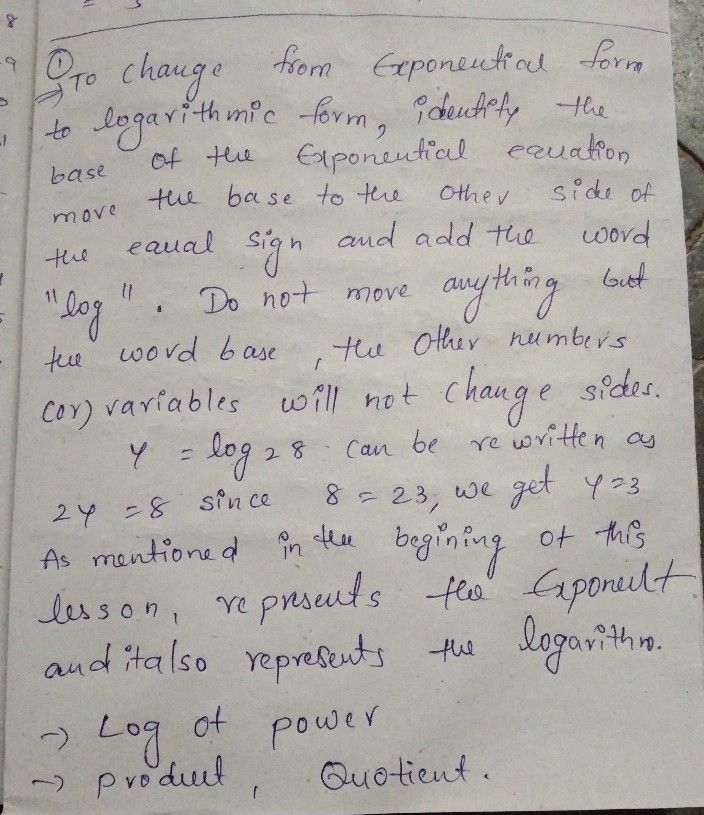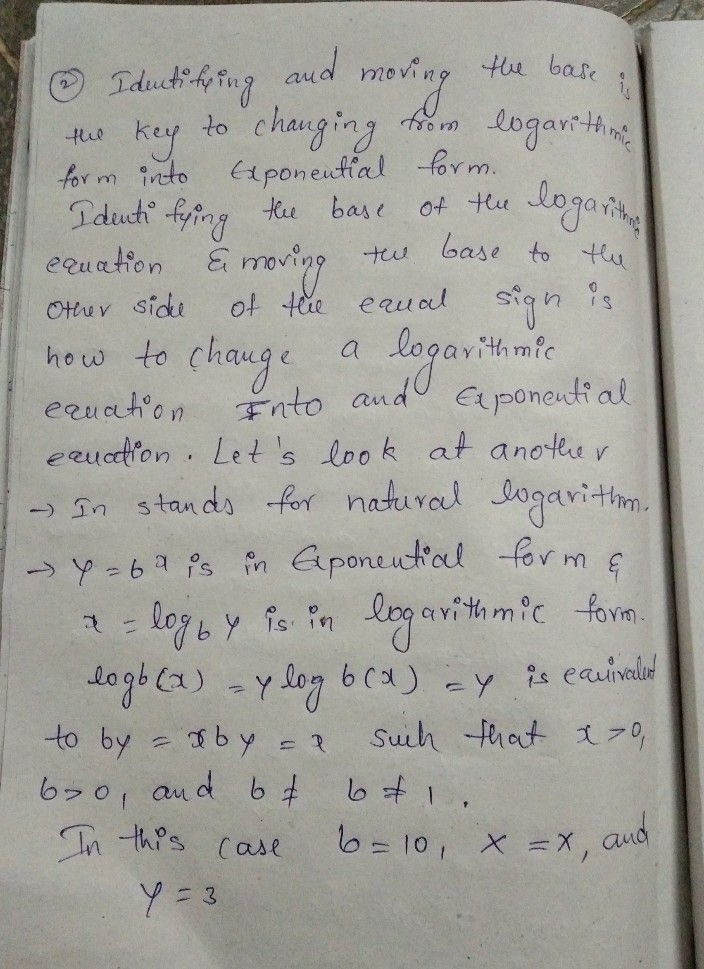Symbol
Problem$1$ How will you rewrite exponential equation to logarithmic form and vice versa? $70$ change exponential equation $10$ logarithmic form, $1$ need $\left(0$ $7$ To change logarithmic equation to exponential form, $1$ need to
10th-13th grade
Calculus
Search count: 116
SolutionQanda teacher - sathish